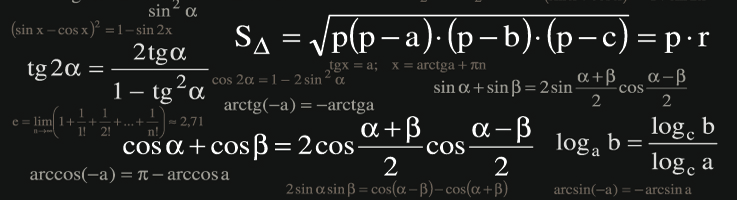# Calculus

In order to excel at Calculus a student needs a strong foundation in Algebra and Trigonometry. Also Calculus books can be extremely conceptual and don’t always present the material in an easy to understand way. This is why tutoring can be so helpful for students who are struggling in class. We can go back and refresh on past math skills or clarify what’s being covered.

#### General Overview of Calculus AB Content

(Content varies across curricula.)

• Essential Functions & Ways to Represent Them
• Inverse Functions, Exponential & Logarithmic Functions

• Finding Limits Graphically & Numerically
• Evaluating Limits Analytically
• Continuity, Limit Laws
• One Sided limits, Limits at Infinity

• Derivatives & Rates of Change
• Basic Differentiation Rules
• Product Rule, Quotient Rule, Chain Rule, Higher Order Derivatives
• Derivatives of Trig Functions & Log Functions
• Implicit Differentiation

• Rates of Change in Natural & Social Sciences
• Related Rates
• Linear Approximation & Differentials
• Hyperbolic Functions

• Extrema on an Interval
• Rolle’s Theorem & the Mean Value Theorem
• Increasing & Decreasing Functions & the First Derivative Test
• Concavity & the Second Derivative Test
• Summary of Curve Sketching

• Indeterminate Forms & l’Hospital’s Rule
• Optimization Problems
• Newton’s Method
• Antiderivatives

• Areas & Distances
• Riemann Sums & The Definite Integral
• The Fundamental Theorem of Calculus
• Indefinite Integrals & the Net Change Theorem
• The Substitution Rule